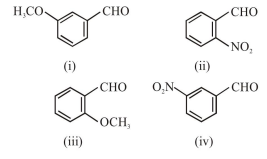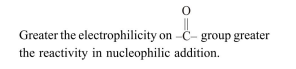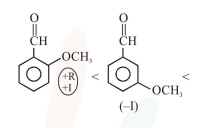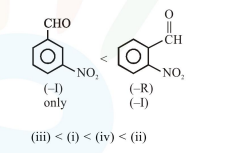# The increasing order of the following compounds towards HCN addition is :

Question:

The increasing order of the following compounds towards HCN addition is :1. (iii) $<$ (iv) $<$ (ii) $<$ (i)

2. (iii) $<$ (iv) $<$ (i) $<$ (ii)

3. (iii) $<$ (i) $<$ (iv) $<$ (ii)

4. (i) $<$ (iii) $<$ (iv) $<$ (ii)

Correct Option: , 3

Solution:

Increasing order of reactivity towards $\mathrm{HCN}$ addition